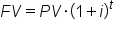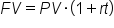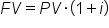+

# Future Value, Single Cash Flows

Author: Sophia Tutorial
##### Description:

Calculate the future value of a single-period or multi-period investment with simple or compound interest rates.

(more)### Developing Effective Teams

*No strings attached. This college course is 100% free and is worth 1 semester credit.

37 Sophia partners guarantee credit transfer.

299 Institutions have accepted or given pre-approval for credit transfer.

* The American Council on Education's College Credit Recommendation Service (ACE Credit®) has evaluated and recommended college credit for 32 of Sophia’s online courses. Many different colleges and universities consider ACE CREDIT recommendations in determining the applicability to their course and degree programs.

Tutorial

what's covered
In this lesson, you will examine how to calculate the future value of a single-period investment and of a multi-period investment with simple and compound interest rates. Specifically, this lesson will cover:
1. Single-Period Investment
2. Multi-Period Investment
1. Simple Interest
2. Compound Interest
3. Calculating Future Value

## 1. Single-Period Investment

The amount of time between the present and future is called the number of periods. A period is a general block of time. Usually, a period is one year. The number of periods can be represented as either t or n.

Suppose you are making an investment, such as depositing your money in a bank. If you plan on leaving the money there for one year, you’re making a single-period investment.

You can calculate an investment with the following formulas:

formula

Compound InterestSimple InterestIn each equation, the variables are defined as:
• FV = Future Value
• PV = Present Value
• i or r = Interest Rate
• t or n = Number of Periods
hint
For all formulas, express interest in decimal form, not as a whole number. 7% is 0.07, 12% is 0.12, and so on.

We will address these formulas later, but note that for a single period of one year, when t = 1, both formulas become FV = PV ⋅ (1+i).

Thus, in a single period, there is only one formula you need to know:

formula
Single-Period Interest### IN CONTEXT

Suppose you deposit \$100 into a bank account that pays 3% interest. What is the balance in your account after one year?

In this case, your PV is \$100 and your interest is 3%. You want to know the value of your investment in the future, so you’re solving for FV. Since this is a single-period investment, t (or n) is 1.

Plugging the numbers into the formula, you get:Your balance will be \$103 in one year.
terms to know

Periods (t or n)
The length of time during which interest accrues, usually one year.
Single-Period Investment
An investment that takes place over one period, usually one year.

## 2. Multi-Period Investment

Multi-period investments take place over more than one period, usually multiple years. They can either accrue simple or compound interest. There are two primary ways of determining how much an investment will be worth in the future if the time frame is more than one period.

terms to know

Multi-Period Investment
An investment that takes place over more than one period.
Accrue
2a. Simple Interest
The first concept of accruing or earning interest is called simple interest. Simple interest means that you earn interest only on the amount you originally invested, or the principal. Your total balance will go up each period, because you earn interest each period, but the interest is paid only on the amount you originally borrowed/deposited.

Simple interest is expressed through the following formula:

formula
Simple Interest### IN CONTEXT

Suppose you make a deposit of \$100 in the bank and earn 5% interest per year. After one year, you earn 5% interest, or \$5, bringing your total balance to \$105. One more year passes, and it’s time to accrue more interest. Since simple interest is paid only on your principal (\$100), you earn 5% of \$100, not 5% of \$105. That means you earn another \$5 in the second year, and will earn \$5 for every year of the investment.

big idea
In simple interest, you earn interest based on the original deposit amount, not the account balance.

term to know

Principal
The money originally invested or loaned, on which basis interest and returns are calculated.
2b. Compound Interest
The second way of accruing interest is called compound interest. In this case, interest is paid at the end of each period based on the balance in the account. In simple interest, it is only how much the principal is that matters. In compound interest, it is what the balance is that matters. Compound interest is named as such because the interest compounds: interest is paid on interest.

Compound interest is expressed through the following formula:

formula
Compound Interest### IN CONTEXT

Suppose you make the same \$100 deposit into a bank account that pays 5%, but this time, the interest is compounded. After the first year, you will again have \$105. At the end of the second year, you also earn 5%, but it’s 5% of your balance, or \$105. You earn \$5.25 in interest in the second year, bringing your balance to \$110.25. In the third year, you earn interest of 5% of your balance, or \$110.25. You earn \$5.51 in interest, bringing your total to \$115.76.

Compare compound interest to simple interest. Simple interest earns you 5% of your principal each year, or \$5 a year. Your balance will go up linearly each year. Compound interest earns you \$5 in the first year, \$5.25 in the second, a little more in the third, and so on. Your balance will go up exponentially.

hint
Simple interest is rarely used compared to compound interest, but it’s good to know both types.

## 3. Calculating Future Value

When calculating a future value (FV), you are calculating how much a given amount of money today will be worth some time in the future. In order to calculate the FV, the other three variables (present value, interest rate, and number of periods) must be known.

hint
Recall that the interest rate is represented by either r or i, and the number of periods is represented by either t or n.

It is also important to remember that the interest rate and the periods must be in the same units. That is, if the interest rate is 5% per year, one period is one year. However, if the interest rate is 5% per month, t or n must reflect the number of periods in terms of months.

### IN CONTEXT

Example 1: Compound, Yearly Loan, Yearly Interest

What is the FV of a \$500, 10-year loan with 7% annual interest?

In this case, the PV is \$500, t is 10 years, and i is 7% per year. The next step is to plug these numbers into an equation. But recall that there are two different formulas for the two different types of interest, simple interest and compound interest. If the problem doesn’t specify how the interest is accrued, assume it is compound interest, at least for business problems.

So, using the compound interest formula, we see that the future value is equal to \$983.58:In practical terms, you just calculated how much your loan will be in 10 years. This assumes that you don’t need to make any payments during the 10 years, and that the interest compounds. Unless the problem states otherwise, it is safe to make these assumptions – you will be told if there are payments during the 10-year period or if it is simple interest.

### IN CONTEXT

Example 2: Compound, Yearly Loan, Monthly Interest

Suppose we want to again find the future value of a \$800, 10-year loan, but with an interest rate of 1% per month.

Remember that the interest rate and periods must be in the same units. In this case, the loan is in units of years, but the interest rate is in units of months. In order to get our total number of periods (t), we would multiply 12 months by 10 years, which equals 120 periods.

Therefore, we can see that the future value is equal to \$2,640.31:### IN CONTEXT

Example 3: Simple, Yearly Loan, Yearly Interest

Suppose you take out a \$5000, eight-year loan, with this one accruing 5% interest per year. The loan accrues interest on the principal only. What is the total future value?

Since the loan is accruing interest on just the principal, this means that we will use the simple interest formula and get a future value of \$7,000:summary
In this lesson, you learned that the future value of a single-period investment can be calculated by knowing the present value and the interest rate. Calculating a multi-period investment involves knowing the present value, the interest rate, the number of time periods, and whether the interest is simple or compounding. Calculating future value is useful for knowing what a given amount of money will be worth at a later date.

Best of luck in your learning!

Source: THIS CONTENT HAS BEEN ADAPTED FROM LUMEN LEARNING'S “Future Value, Single Amount” TUTORIAL.

Terms to Know
Accrue

Multi-Period Investment

An investment that takes place over more than one periods.

Periods (t or n)

The length of time during which interest accrues, usually one year.

Principal

The money originally invested or loaned, on which basis interest and returns are calculated.

Single-Period Investment

An investment that takes place over one period, usually one year.

Formulas to Know
Compound InterestSimple InterestSingle-Period InterestRating